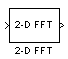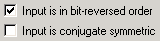Documentation

2-D FFT

Compute two-dimensional fast Fourier transform of input

Library

Transforms

visiontransformsDescription

The 2-D FFT block computes the fast Fourier transform (FFT). The block does the computation of a two-dimensional M-by-N input matrix in two steps. First it computes the one-dimensional FFT along one dimension (row or column). Then it computes the FFT of the output of the first step along the other dimension (column or row).

The output of the 2-D FFT block is equivalent to the MATLAB® fft2 function:

y = fft2(A)	% Equivalent MATLAB code

Computing the FFT of each dimension of the input matrix is equivalent to calculating the two-dimensional discrete Fourier transform (DFT), which is defined by the following equation:

$F\left(m,n\right)=\sum _{x=0}^{M-1}\sum _{y=0}^{N-1}f\left(x,y\right){e}^{-j\frac{2\pi mx}{M}}{e}^{-j\frac{2\pi ny}{N}}$

where $0\le m\le M-1$ and $0\le n\le N-1$.

The output of this block has the same dimensions as the input. If the input signal has a floating-point data type, the data type of the output signal uses the same floating-point data type. Otherwise, the output can be any fixed-point data type. The block computes scaled and unscaled versions of the FFT.

The input to this block can be floating-point or fixed-point, real or complex, and conjugate symmetric. The block uses one of two possible FFT implementations. You can select an implementation based on the FFTW library , , or an implementation based on a collection of Radix-2 algorithms. You can select Auto to allow the block to choose the implementation.

Port Description

PortDescriptionSupported Data TypesComplex Values Supported

Input

Vector or matrix of intensity values

• Double-precision floating point

• Single-precision floating point

• Fixed point

• 8-, 16-, 32-bit signed integer

• 8-, 16-, 32-bit unsigned integer

Yes

Output

2-D FFT of the input

Same as Input port

Yes

FFTW Implementation

The FFTW implementation provides an optimized FFT calculation including support for power-of-two and non-power-of-two transform lengths in both simulation and code generation. Generated code using the FFTW implementation will be restricted to those computers which are capable of running MATLAB. The input data type must be floating-point.

The Radix-2 implementation supports bit-reversed processing, fixed or floating-point data, and allows the block to provide portable C-code generation using the Simulink Coder. The dimensions of the input matrix, M and N, must be powers of two. To work with other input sizes, use the Image Pad block to pad or truncate these dimensions to powers of two, or if possible choose the FFTW implementation.

With Radix-2 selected, the block implements one or more of the following algorithms:

• Butterfly operation

• Double-signal algorithm

• Half-length algorithm

• Radix-2 decimation-in-time (DIT) algorithm

• Radix-2 decimation-in-frequency (DIF) algorithm

Radix-2 Algorithms for Real or Complex Input Complexity Floating-Point Signals

Other Parameter Settings

Algorithms Used for IFFT ComputationButterfly operation and radix-2 DITButterfly operation and radix-2 DIT in conjunction with the half-length and double-signal algorithmsRadix-2 DIF in conjunction with the half-length and double-signal algorithms

Radix-2 Algorithms for Real or Complex Input Complexity Fixed-Point Signals

Other Parameter Settings

Algorithms Used for IFFT ComputationButterfly operation and radix-2 DITNote

The Input is conjugate symmetric parameter cannot be used for fixed-point signals.

Radix-2 Optimization for the Table of Trigonometric Values

In certain situations, the block’s Radix–2 algorithm computes all the possible trigonometric values of the twiddle factor

${e}^{j\frac{2\pi k}{K}}$

where K is the greater value of either M or N and $k=0,\cdots ,K-1$. The block stores these values in a table and retrieves them during simulation. The number of table entries for fixed-point and floating-point is summarized in the following table:

Number of Table Entries for N-Point FFT

floating-point

3 N/4

fixed-point

N

Fixed-Point Data Types

The following diagrams show the data types used in the FFT block for fixed-point signals. You can set the sine table, accumulator, product output, and output data types displayed in the diagrams in the FFT dialog box as discussed in Parameters.

Inputs to the FFT block are first cast to the output data type and stored in the output buffer. Each butterfly stage then processes signals in the accumulator data type, with the final output of the butterfly being cast back into the output data type. The block multiplies in a twiddle factor before each butterfly stage in a decimation-in-time FFT and after each butterfly stage in a decimation-in-frequency FFT.The multiplier output appears in the accumulator data type because both of the inputs to the multiplier are complex. For details on the complex multiplication performed, refer to Multiplication Data Types.

Parameters

FFT implementation

Set this parameter to FFTW ,  to support an arbitrary length input signal. The block restricts generated code with FFTW implementation to host computers capable of running MATLAB.

Set this parameter to Radix-2 for bit-reversed processing, fixed or floating-point data, or for portable C-code generation using the Simulink Coder. The dimensions of the input matrix, M and N, must be powers of two. To work with other input sizes, use the Image Pad block to pad or truncate these dimensions to powers of two, or if possible choose the FFTW implementation. See Radix-2 Implementation.

Set this parameter to Auto to let the block choose the FFT implementation. For non-power-of-two transform lengths, the block restricts generated code to MATLAB host computers.

Output in bit-reversed order

Designate the order of the output channel elements relative to the ordering of the input elements. When you select this check box, the output channel elements appear in bit-reversed order relative to the input ordering. If you clear this check box, the output channel elements appear in linear order relative to the input ordering.

Linearly ordering the output requires extra data sorting manipulation. For more information, see Bit-Reversed Order.

Scale result by FFT length

When you select this parameter, the block divides the output of the FFT by the FFT length. This option is useful when you want the output of the FFT to stay in the same amplitude range as its input. This is particularly useful when working with fixed-point data types.

Rounding mode

Select the Rounding Modes for fixed-point operations. The sine table values do not obey this parameter; instead, they always round to Nearest.

Saturate on integer overflow

Select the overflow mode for fixed-point operations. See Precision and Range. The sine table values do not obey this parameter; instead, they are always saturated.

Sine table data type

Choose how you specify the word length of the values of the sine table. The fraction length of the sine table values always equals the word length minus one. You can set this parameter to:

• A rule that inherits a data type, for example, Inherit: Same word length as input

• An expression that evaluates to a valid data type, for example, fixdt(1,16)

The sine table values do not obey the Rounding mode and Saturate on integer overflow parameters; instead, they are always saturated and rounded to Nearest.

Product output data type

Specify the product output data type. See Fixed-Point Data Types and Multiplication Data Types for illustrations depicting the use of the product output data type in this block. You can set this parameter to:

• A rule that inherits a data type, for example, Inherit: Inherit via internal rule

• An expression that evaluates to a valid data type, for example, fixdt(1,16,0)

Click the buttonto display the Data Type Assistant, which helps you set the Product output data type parameter.

See Specify Data Types Using Data Type Assistant (Simulink) for more information.

Accumulator data type

Specify the accumulator data type. See Fixed-Point Data Types for illustrations depicting the use of the accumulator data type in this block. You can set this parameter to:

• A rule that inherits a data type, for example, Inherit: Inherit via internal rule

• An expression that evaluates to a valid data type, for example, fixdt(1,16,0)

Click the buttonto display the Data Type Assistant, which helps you set the Accumulator data type parameter.

See Specify Data Types Using Data Type Assistant (Simulink) for more information.

Output data type

Specify the output data type. See Fixed-Point Data Types for illustrations depicting the use of the output data type in this block. You can set this parameter to:

• A rule that inherits a data type, for example, Inherit: Inherit via internal rule.

When you select Inherit: Inherit via internal rule, the block calculates the output word length and fraction length automatically. The internal rule first calculates an ideal output word length and fraction length using the following equations:

• When you select the Divide butterfly outputs by two check box, the ideal output word and fraction lengths are the same as the input word and fraction lengths.

• When you clear the Divide butterfly outputs by two check box, the block computes the ideal output word and fraction lengths according to the following equations:

$W{L}_{idealoutput}=W{L}_{input}+floor\left({\mathrm{log}}_{2}\left(FFTlength-1\right)\right)+1$

$F{L}_{idealoutput}=F{L}_{input}$

Using these ideal results, the internal rule then selects word lengths and fraction lengths that are appropriate for your hardware. For more information, see Inherit via Internal Rule.

• An expression that evaluates to a valid data type, for example, fixdt(1,16,0)

Click the buttonto display the Data Type Assistant, which helps you set the Output data type parameter.

See Specify Data Types Using Data Type Assistant (Simulink) for more information.

Lock data type settings against change by the fixed-point tools

Select this parameter to prevent the fixed-point tools from overriding the data types you specify on the block mask. For more information, see fxptdlg, a reference page on the Fixed-Point Tool in the Simulink® documentation.

Example

Bit-Reversed Order

Two numbers are bit-reversed values of each other when the binary representation of one is the mirror image of the binary representation of the other. For example, in a three-bit system, one and four are bit-reversed values of each other because the three-bit binary representation of one, 001, is the mirror image of the three-bit binary representation of four, 100. The following diagram shows the row indices in linear order. To put them in bit-reversed order

1. Translate the indices into their binary representation with the minimum number of bits. In this example, the minimum number of bits is three because the binary representation of 7 is 111.

2. Find the mirror image of each binary entry, and write it beside the original binary representation.

3. Translate the indices back to their decimal representation.

The row indices now appear in bit-reversed order.If, on the 2-D FFT block parameters dialog box, you select the Output in bit-reversed order check box, the block bit-reverses the order of both the columns and the rows. The next diagram illustrates the linear and bit-reversed outputs of the 2-D FFT block. The output values are the same, but they appear in different order.References

 Frigo, M. and S. G. Johnson, “FFTW: An Adaptive Software Architecture for the FFT,”Proceedings of the International Conference on Acoustics, Speech, and Signal Processing, Vol. 3, 1998, pp. 1381-1384.